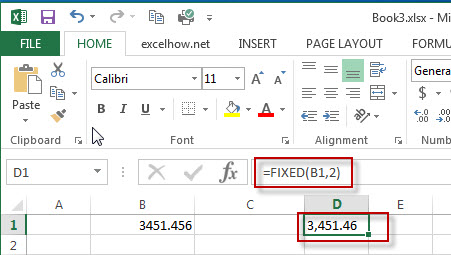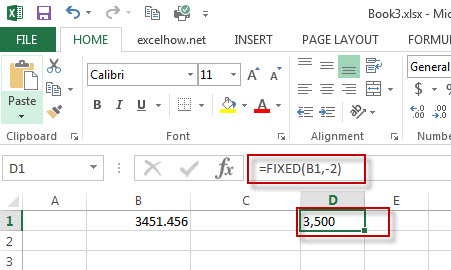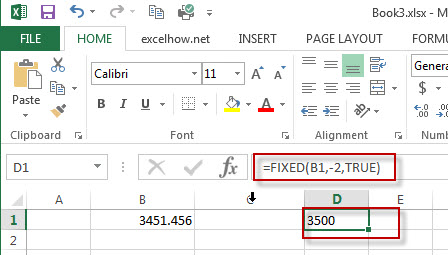# Excel Fixed Function

This post will guide you how to use Excel FIXED function with syntax and examples in Microsoft excel.

### Description

The Excel FIXED function rounds a number to the specified number of decimal places and returns the result as text string.

The FIXED function is a build-in function in Microsoft Excel and it is categorized as a Text Function.

The FIXED function is available in Excel 2016, Excel 2013, Excel 2010, Excel 2007, Excel 2003, Excel XP, Excel 2000, Excel 2011 for Mac.

### Syntax

The syntax of the FIXED function is as below:

= FIXED(number, [decimals],[no_commas])

Where the FIXED function arguments are:
number -This is a required argument. The number to be rounded and converted into text string.
Decimals-This is an optional argument. It will specify the number of decimal places to be displayed after decimal point.
No_commas– This is an optional argument.  This is a logical argument. If it is set to TRUE, the result will not separate number groups by commas, or commas are included in the result text string. (If this argument is omitted, the result text string will include commas as default)

Note: If Decimals value is omitted, it is set to be 2 by default.  If Decimals value is negative, the number is rounded to the left of the decimal point.

### Example

The below examples will show you how to use Excel FIXED Text function to round a number to the specified number of decimal places.

#1 To round the number in B1 cell two digit to the right of the decimal point, just using FIXED(B1,2) formulas.#2 To round the number in B2 cell two digit to the left of the decimal point, just using FIXED(B1,-2) formulas.#3 To round the number in B3 cell two digit to the left of the decimal point without commas, just using FIXED(B1,-2,TRUE) formulas.